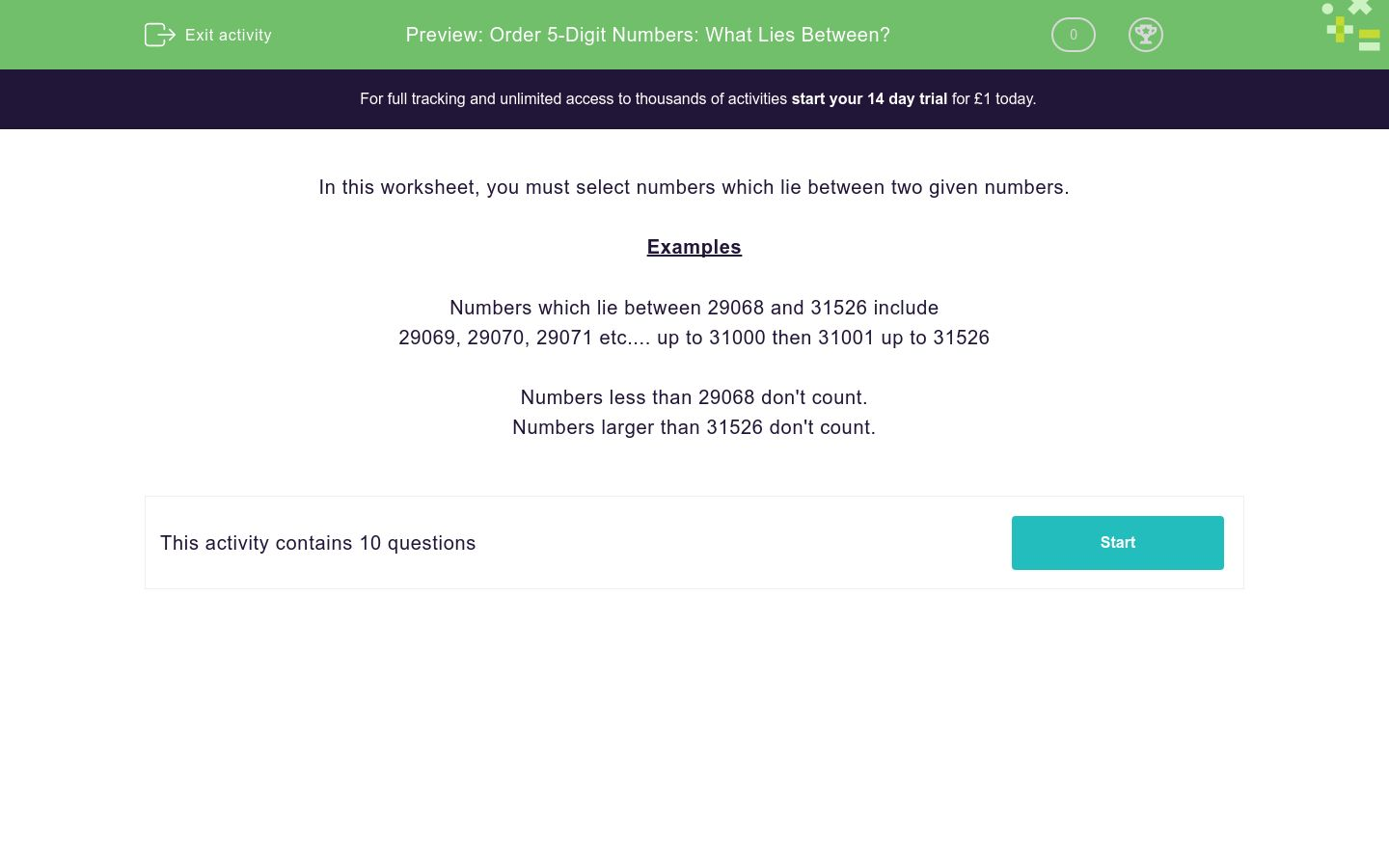# Order 5-Digit Numbers: What Lies Between?

In this worksheet, students select any numbers that lie between two given numbers.Key stage:  KS 2

Curriculum topic:   Number: Number and Place Value

Curriculum subtopic:   Know Numbers to 1 000 000

Difficulty level:### QUESTION 1 of 10

In this worksheet, you must select numbers which lie between two given numbers.

Examples

Numbers which lie between 29068 and 31526 include

29069, 29070, 29071 etc.... up to 31000 then 31001 up to 31526

Numbers less than 29068 don't count.

Numbers larger than 31526 don't count.

Select the numbers which lie between these two numbers.

81068 and 90526

81069

87559

54689

46856

98525

Select the number(s) which lie between these two numbers.

91238 and 99526

81069

87559

91529

46856

115025

Select the number(s) which lie between these two numbers.

29068 and 31526

26564

87559

29658

46856

54686

Select the number(s) which lie between these two numbers.

27545 and 31526

26564

87559

29658

46856

54686

Select the number(s) which lie between these two numbers.

59068 and 91840

26564

87559

29658

91838

54686

Select the number(s) which lie between these two numbers.

36300 and 46526

26564

87559

29658

36341

45637

Select the number(s) which lie between these two numbers.

29068 and 56526

56533

55260

29658

36341

53265

Select the number(s) which lie between these two numbers.

71500 and 72500

72319

55260

79796

36341

71921

Select the number(s) which lie between these two numbers.

55000 and 66000

72319

55260

79796

65441

65440

Select the number(s) which lie between these two numbers.

45394 and 46000

85571

55588

45395

65441

65440

• Question 1

Select the numbers which lie between these two numbers.

81068 and 90526

81069
87559
EDDIE SAYS
Look at the numbers in the ten thousands and thousands place to be sure they are in the same set between 81068 and 90526.
• Question 2

Select the number(s) which lie between these two numbers.

91238 and 99526

91529
EDDIE SAYS
Look at the numbers in the ten thousands and thousands place to be sure they are in the same set between 91238 and 99526.
• Question 3

Select the number(s) which lie between these two numbers.

29068 and 31526

29658
EDDIE SAYS
Look at the numbers in the ten thousands and thousands place to be sure they are in the same set between 29068 and 31526.
• Question 4

Select the number(s) which lie between these two numbers.

27545 and 31526

29658
EDDIE SAYS
Look at the numbers in the ten thousands and thousands place to be sure they are in the same set between 27545 and 31526.
• Question 5

Select the number(s) which lie between these two numbers.

59068 and 91840

87559
91838
EDDIE SAYS
Look at the numbers in the ten thousands and thousands place to be sure they are in the same set between 59068 and 91840.
• Question 6

Select the number(s) which lie between these two numbers.

36300 and 46526

36341
45637
EDDIE SAYS
Look at the numbers in the ten thousands and thousands place to be sure they are in the same set between 36300 and 46526.
• Question 7

Select the number(s) which lie between these two numbers.

29068 and 56526

55260
29658
36341
53265
EDDIE SAYS
Look at the numbers in the ten thousands and thousands place to be sure they are in the same set between 29068 and 56526.
• Question 8

Select the number(s) which lie between these two numbers.

71500 and 72500

72319
71921
EDDIE SAYS
Look at the numbers in the ten thousands and thousands place to be sure they are in the same set between 71500 and 72500.
• Question 9

Select the number(s) which lie between these two numbers.

55000 and 66000

55260
65441
65440
EDDIE SAYS
Look at the numbers in the ten thousands and thousands place to be sure they are in the same set between 55000 and 66000.
• Question 10

Select the number(s) which lie between these two numbers.

45394 and 46000

45395
EDDIE SAYS
Look at the numbers in the ten thousands and thousands place to be sure they are in the same set between 45394 and 46000.
---- OR ----

Sign up for a £1 trial so you can track and measure your child's progress on this activity.

### What is EdPlace?

We're your National Curriculum aligned online education content provider helping each child succeed in English, maths and science from year 1 to GCSE. With an EdPlace account you’ll be able to track and measure progress, helping each child achieve their best. We build confidence and attainment by personalising each child’s learning at a level that suits them.

Get started